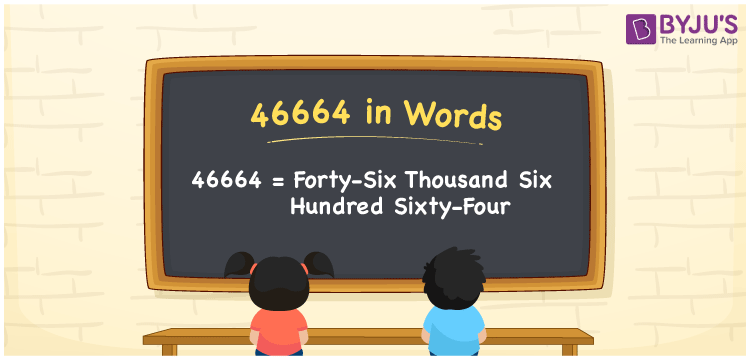# 46664 in Words

46664 in words is written as Forty-six thousand six hundred sixty-four. In both the International System of Numerals and the Indian System of Numerals, 46664 is written as Forty-six thousand six hundred sixty-four. The number 46664 is a Cardinal Number as it represents some quantity. For example, “the population of this town is about 46664”.

 46664 in Words Forty-six thousand six hundred sixty-four Forty-six thousand six hundred sixty-four in Number 46664

## 46664 in English Words

46664 in English words is read as “Forty-six thousand six hundred sixty-four”.## How to Write 46664 in Words?

To write 46664 in words, we shall use the place value chart. In the place value chart, put 4 in the ten thousands, 6 in the thousands, 6 in the hundreds, 6 in the tens and 4 in the ones. Let us make a place value chart to write the number 46664 in words.

 Ten Thousands Thousands Hundreds Tens Ones 4 6 6 6 4

Thus, we can write the expanded form as

4 × Ten Thousand + 6 × Thousand + 6 × Hundred + 6 × Ten + 4 × One

= 4 × 10000 + 6 × 1000 + 6 × 100 + 6 × 10 + 4 × 1

= 40000 + 6000 + 600 + 60 + 4

= 46664

= Forty-six thousand six hundred sixty-four.

46664 is a natural number, the successor of 46663 and the predecessor of 46665.

46664 in words – Forty-six thousand six hundred sixty-four

• Is 46664 an odd number? – No
• Is 46664 an even number? – Yes
• Is 46664 a perfect square number? – No
• Is 46664 a perfect cube number? – No
• Is 46664 a prime number? – No
• Is 46664 a composite number? – Yes

## Frequently Asked Questions on 46664 in Words

Q1

### How to write 46664 in words?

46664 in words is written as Forty-six thousand six hundred sixty-four.
Q2

### How to write 46664 in the International and Indian System of Numerals?

In both, the system of numerals, 46664 in words, is written as Forty-six thousand six hundred sixty-four.
Q3

### How to write 46664 in a place value chart?

In the place value chart, write 4 in the ten thousands, 6 in the thousands, 6 in the hundreds, 6 in the tens and 4 in the ones, respectively.